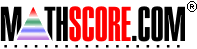Math Practice Online > free > lessons > Florida > 6th grade > Area And Volume Proportions

## Area And Volume Proportions

This topic deals with understanding how changing certain sides changes area and volume.

 Sample Problems for Area And Volume Proportions Lesson for Area And Volume Proportions

### This topic aligns to the following state standards

Grade 5: Geo 3. Knows how area and perimeter are affected when geometric figures are combined, rearranged, enlarged, or reduced (for example, What happens to the area of a square when the sides are doubled?).
Grade 6: Meas 2. Knows the relationship between the area or perimeter of an original figure and that of a newly created figure.
Grade 6: Meas 3. Solves real-world or mathematical problems involving perimeter or area and how these are affected by changes in the dimensions of the figure.
Grade 7: Meas 2. Knows the relationships between the perimeters, areas, surface areas, or volumes of the original figure and those of the newly created figure.
Grade 7: Meas 3. Solves real world or mathematical problems involving perimeter, area, circumference, surface area and volume and how these are affected by changes in the dimensions of the figures.
Grade 8: Meas 1. Knows how a change in a figure's dimensions affects its perimeter, area, circumference, surface area, or volume.
Grade 8: Meas 3. Solves real-world or mathematical problems involving the effects of changes either to the dimensions of a figure or to the volume, surface area, area, perimeter, or circumference of figures.

Copyright Accurate Learning Systems Corporation 2008.